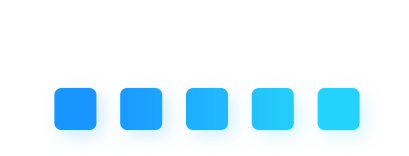\u200E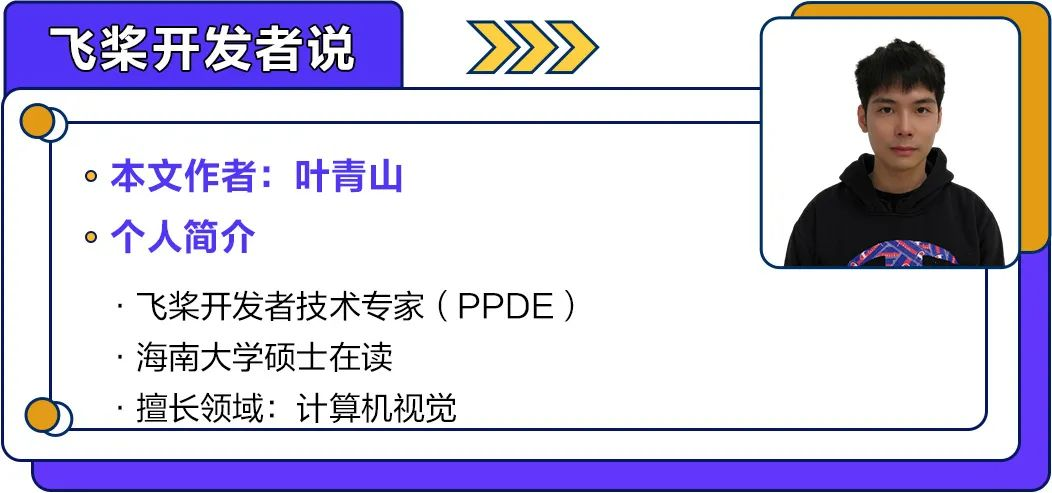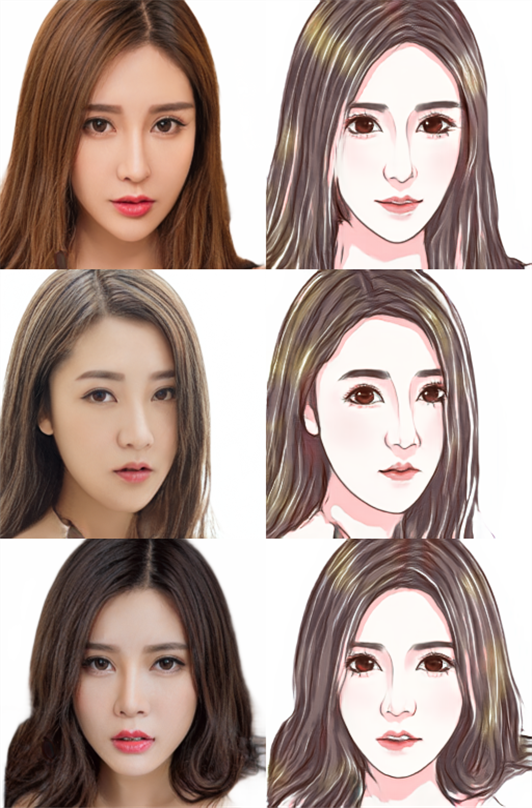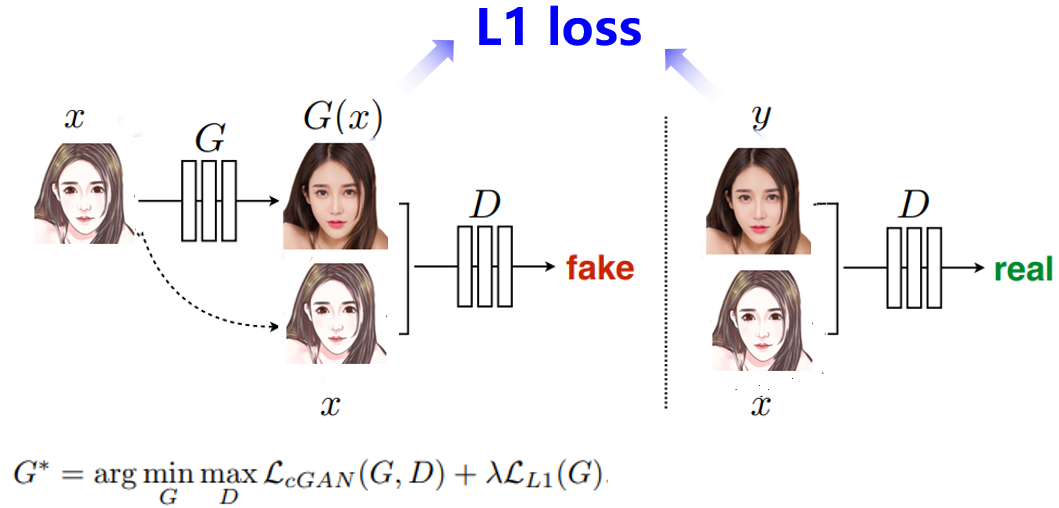X:原图   G(X):生成图  G:生成器   D:判别器  Y:标签

1.数据准备及预处理

• 数据类别：动漫照片（原图）/对应的真人图片（标签）。
• 数据数量：共计约1400张图像。
• 数据划分：训练集、测试集中的比例13:1。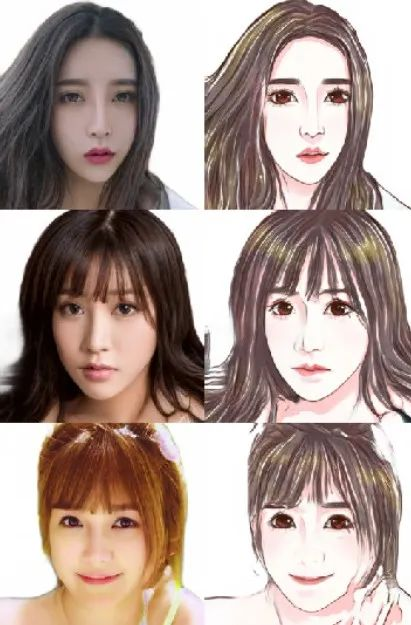2.自定义生成器和判别器

``generator = UnetGenerator()discriminator = NLayerDiscriminator()out = generator(paddle.ones([1, 3, 256, 256]))print('生成器输出尺寸：', out.shape)out = discriminator(paddle.ones([1, 6, 256, 256]))print('判别器输出尺寸：', out.shape)输出结果如下：生成器输出尺寸： [1, 3, 256, 256]判别器输出尺寸： [1, 1, 30, 30]``
`实例化生成器、判别器`

3.定义超参数进行训练

``# 超参数LR = 1e-4BATCH_SIZE = 8EPOCHS = 100# 优化器optimizerG = paddle.optimizer.Adam(    learning_rate=LR,    parameters=generator.parameters(),    beta1=0.5,    beta2=0.999)optimizerD = paddle.optimizer.Adam(    learning_rate=LR,    parameters=discriminator.parameters(),     beta1=0.5,    beta2=0.999)# 损失函数bce_loss = nn.BCELoss()l1_loss = nn.L1Loss()``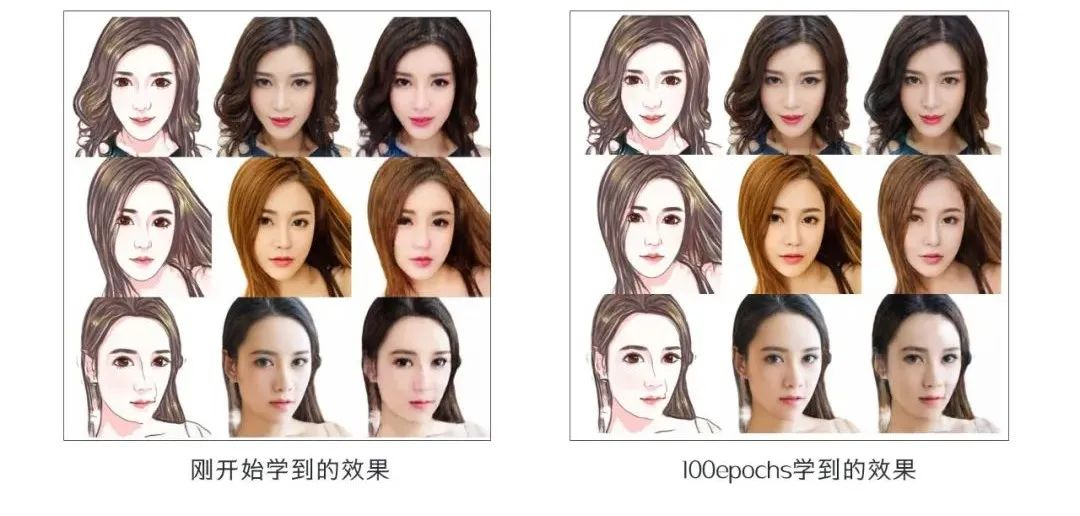4.测试

``# 为生成器加载权重weights_save_path = 'work/weights'last_weights_path = os.path.join(weights_save_path, sorted(os.listdir(weights_save_path))[-1])print('加载权重:', last_weights_path)model_state_dict = paddle.load(last_weights_path)generator.load_dict(model_state_dict)generator.eval()# 读取数据# test_names = os.listdir('data/cartoon_A2B/test')# # img_name = np.random.choice(test_names)img_name = '01481.png'img_A2B = cv2.imread('data/cartoon_A2B/test/'+img_name)img_A = img_A2B[:, 256:]                                  # 卡通图（即输入）img_B = img_A2B[:, :256]                                  # 真人图（即预测结果）``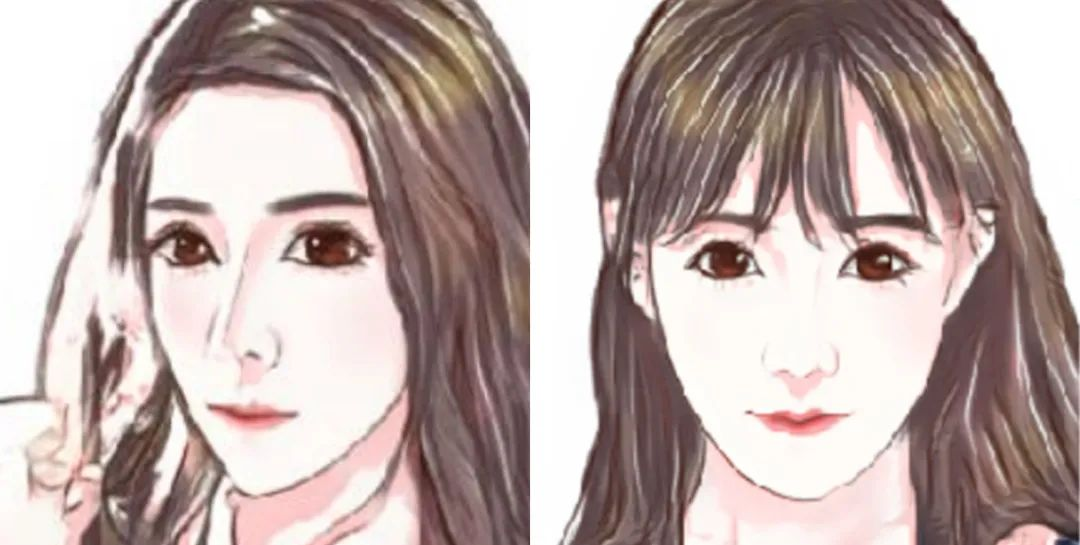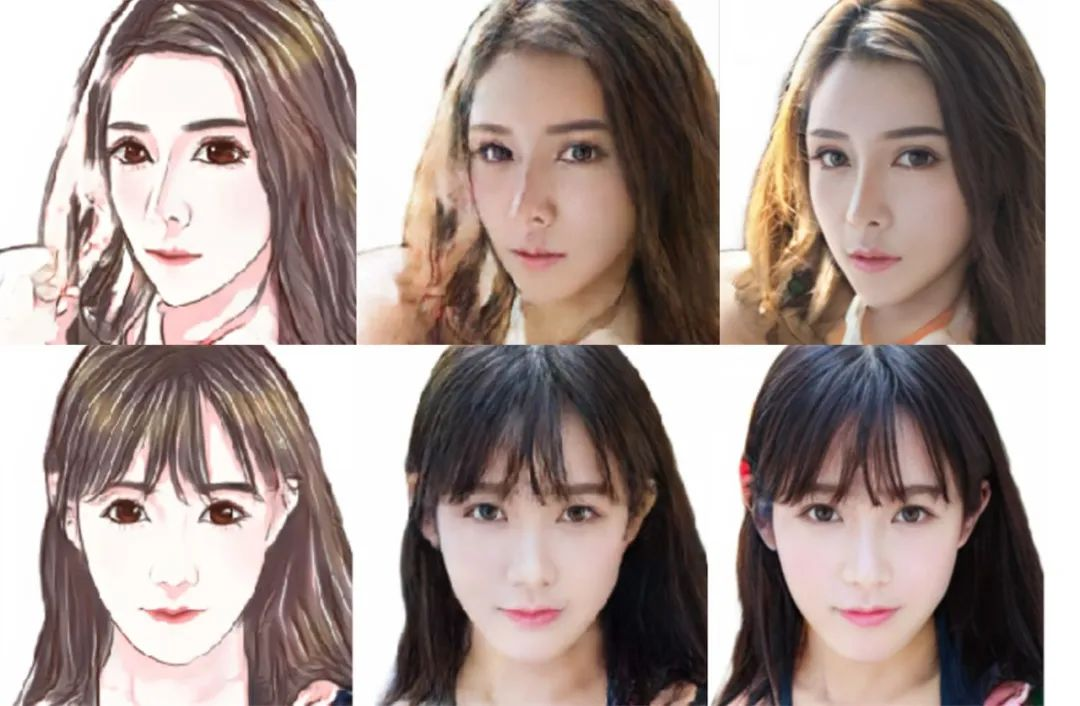• 数据集风格较为单一（特征简单），使用不同动漫风格的照片进行图像生成，可能会导致生成效果较差。这是因为模型泛化性能仍存在优化空间。另外，目前本项目所采用的数据集只有女性一种性别，无法对男性进行生成图像。
• 原图阴影部分对应生成部分效果一般会比较差。此外，也存在光照敏感度不强烈的问题。

• 可以尝试优化数据集，即增加数据集风格种类、性别，并且保持一定数量的增加。

• 可以更换更好的生成器模型，以加强网络学习更多细节的信息。

• 点击阅读原文获得项目链接：
https://aistudio.baidu.com/aistudio/projectdetail/3450122
• 更多趣味体验欢迎访问：